## 2. 4. 1 Geometrical Properties of the Elements

At this point the topological representation of the cell complex is added to the geometrical representation. Whereas the topological information contains which elements are subsets or supersets of other functions, the geometrical properties represent the actual position of the single element.

A typical geometric property is, for instance, the coordinate information of a point or the information, whether a point is contained within a cell. In order to obtain a homeomorphic mapping from the cell complex into the domain of the function, which is usually a partial space of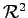or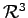or in some cases some (preferably differentiable) manifold, it is required that each element of the tesselation can be defined via a continuous representation in the domain.

As an example, all connection lines between points can be assumed to be linear and all surfaces of an element can be assumed to be plain. In this case it is only necessary to provide the coordinate information of the points in order to explicitly determine the shape of the elements. For instance, a triangle can be defined by giving all its points. Fig. 2.7 shows the separation of topological and geometrical information when using straight edges.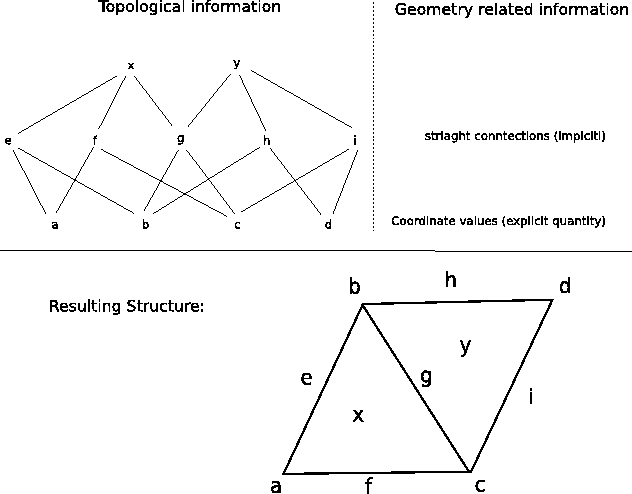The connection line between two given points does not necessarily have to be straight. Any continuous line could be given, which perceives the continuous (or differentiable) mapping from the cell complex to the simulation domain. The same holds true for the specification of surfaces.

It can be arranged that all data related to the geometrical representation of a certain element of the tesselation can be associated with the respective element. As an example, coordinate values of a point are associated to the respective vertex, and curvature information of the edges, such as curvature coefficients, can be associated with the topological edge structures of the cell complex. The main advantage of this method is that the information is stored in a consistent manner, because for the evaluation of geometric data, e.g. the measuring of the volume of each of the two two neighboring cells, it is possible to use the same curvature information of the intersecting surface. In contrast, the association of the surface curvature with the cells possibly leads to a non-unique definition of the bounding surface (see Fig 2.8).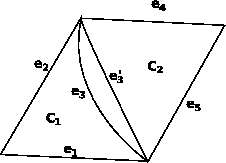In analogy to the shape functions, the geometrical structure of the topologically defined cells is related to some assumptions. The more implicit assumptions are made about the geometrical properties, the less information has to be explicitly stored of the single elements and vice versa.

An example is the specification of simplicial cells (topological definition). A possible geometric definition of such a cell is e.g., a simplex which defines all edges to be straight and all surfaces to be plain. In such a case, the only data required are the coordinates of the points, because all further information of the position of higher order elements can be derived. Implicitly, the following properties are assumed: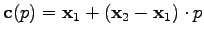(2.29)

In contrast, for each element of the cell, a polynomial (or any other) function can be assumed which represents the shape of the respective element when a set of parameters is given. In the following case, a triangle is used, where the geometrical shape of the edges is represented by parameterization and the parameter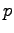is within the unity range, and the pointsand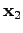denote the point vectors of the bounding vertices and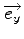anddenote the unit vectors of a given coordinate system.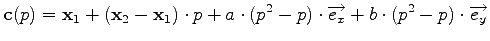(2.30)

The parameters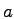and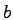denote the actual shape of the edge. If both are zero, the formulation yields a straight edge between the pointsand.

The parameters used in the definition are associated to the lowest-dimensional element for whose definition they are relevant. A set of all incident lower dimensional elements can be obtained via traversal.

The information regarding the position of a point is stored in the respective vertex. If a parametric (geometrical) formulation of an edge, which is incident to the vertex is required, one can obtain the required information from the underlying vertex. However, the geometrical formulation used implicitly has to guarantee, that the topological properties of the elements are preserved under the geometrical transformation.

For this reason it has to be checked, if a higher dimensional element can be based on lower dimensional elements. If, for instance, two points are congruent, the construction of an edge based on these points is obviously not possible. This also implies that the curvature coefficients of curved edges of a triangle must not be too large, in order not to degenerate the triangle. In such a case there is no proper mapping between the topological elements and their geometrical representation.

However, if the information of vertices is given explicitly and the necessary geometrical information to construct a higher-dimensional element from its incident lower dimensional boundary is stored in this element, a consistent geometrical definition of all cells can be given. The information to construct higher-dimensional elements from lower dimensional elements can therefore either be given globally by using straight bounded elements only or by locally defining curvature coefficients.

Michael 2008-01-16